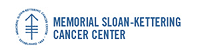## Memorial Sloan-Kettering Cancer Center, Dept. of Epidemiology & Biostatistics Working Paper Series

#### Abstract

Power calculations in a small sample comparative study, with a continuous outcome measure, are typically undertaken using the asymptotic distribution of the test statistic. When the sample size is small, this asymptotic result can be a poor approximation. An alternative approach, using a rank based test statistic, is an exact power calculation. When the number of groups is greater than two, the number of calculations required to perform an exact power calculation is prohibitive. To reduce the computational burden, a Monte Carlo resampling procedure is used to approximate the exact power function of a k-sample rank test statistic under the family of Lehmann alternative hypotheses. The motivating example for this approach is the design of animal studies, where the number of animals per group is typically small.

COinS